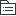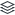竞品维度分析

|
7

相关模板推荐

•竞品维度分析 —— 作品大纲

•市场
• 竞争格局
• 红海
• 蓝海
• 商业模式
• 传统
• 创新
• 垂直
• 一米宽千米深
• 平台
• 内容
• PGC/UGC
• ...
• 市场占有率分布
• top排名产品
• 市场趋势资讯
• 投资人
• 创业者
• 行业媒体资讯
• 36氪
• 创业邦
• 投资界
• ...
•资源
• 团队
• 投资人
• 合作伙伴
• 政府支持
• 既有产品线
• 自研产品
• 配套产品
•用户
• 用户同理心分析
• 用户确定性分析
• 用户画像确定分析
• 用户体验层次分析
• 用户体验地图绘制
• 服务用户蓝图峰值分析
• 用户口碑调查
• 用户行为趋势、数据分析
•产品
• 选择研究对象
• 挑市场重点产品
• 挑比自己更成熟的产品
• 扮演用户
• 小白用户
• 关注第一印象
• 用户盲测
• 功能分析
• 多终端比较
• Android/iOS/wp
• 多商业入口分析
• APP/H5/公总号/小程序/PC
• 版本历史追踪分析
• 功能特色
• UI与交互分析
• 多终端比较
• Android/iOS/wp
• 同类产品比较/新趋势
• 是否符合公司文化/平台使用习惯
• 技术分析
• 如何解决和规避同类产品问题
• 缺点、特点、创新
•运营
• 营销
• 宣传点
• 宣传力度
• 宣传渠道或方式
• 软文
• 硬广告
• 网络影响力
• 媒体曝光
• 搜索引擎
• 微博热度
• 外部链接
• 流量分析
• Alexa
• quantcast
• compete
• 运营时间估算
• 查域名启动时间
• 搜索引擎收录量
• 用户估算
• 访问量
• 转化率
• 运营成本估算
• 人员
• 宽带服务器
• 营销
• 营收状况预估
• 收集公开数据并跟踪
• 合伙人数据调查
•岗位适配
• 了解资源的人
• what
• 竞争对手的资源
• 自己的资源
• who
• 公司CEO/高管
• 其他人员
• 主动收集信息
• 拓宽行业人脉视野
• 关心公司状况
• 能用好资源的人
• 有权利调配资源
• 对投入产品敏感
• 能决定或影响项目/产品决策
• 难度
• 资源不了解
• 资源配备不齐
• 角色太多样
• 市场
• 产品
• 交互
• 技术
• 用户
•处境
• 我做别人也做
• 我想做别人没做
• 我们不做别人在做